# A Note on Boxplots in R

As a statistical consultant I frequently use boxplots. They're a great way to quickly visualize the distribution of a continuous measure by some grouping variable. More often than not, however, the person I'm helping doesn't regularly use boxplots (if at all) and is not sure what to make of them.

Fortunately, boxplots are pretty easy to explain. The line in the middle of the box is the median. The box itself represents the middle 50% of the data. The box edges are the 25th and 75th percentiles. But what about the whiskers? That seems to be the part that trips people up.

Before we go further, let's make some boxplots in R:

set.seed(9)
y <- rnorm(n = 100, mean = rep(c(22:25),each=25),sd = rep(c(5,8),each=50))
x <- gl(n=4,k=25)
boxplot(y ~ x)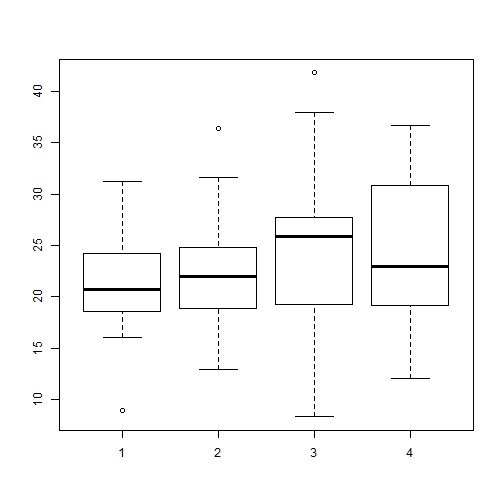Above I generate 100 random normal values, 25 each from four distributions: N(22,5), N(23,5), N(24,8) and N(25,8). Then I generate a 4-level grouping variable. Finally I make the boxplot.

The black lines in the “middle” of the boxes are the median values for each group. For group 1, that appears to be a shade above 20. The vertical size of the boxes are the interquartile range, or IQR. They measure the spread of the data, sort of like standard deviation. The tops and bottoms of the boxes are referred to as “hinges”. We see groups 1 and 2 have less variability than groups 3 and 4, which makes sense given the way we generated the data. The IQR for group 1 looks to be about 5 judging from the height of the box. Again this makes sense given group 1 data was randomly generated from a normal distribution with a standard deviation of 5.

And now we come to the “whiskers”, or the flattened arrows extending out of the box. What do they represent and how are they calculated? They represent the reasonable extremes of the data. That is, these are the minimum and maximum values that do not exceed a certain distance from the middle 50% of the data. What is that certain distance? By default in R, it's $$1.5 \times IQR$$. If no points exceed that distance, then the whiskers are simply the minimum and maximum values. That's the case in group 4. If there are points beyond that distance, the largest point that does not exceed that distance becomes the whisker. And the points beyond the distance are plotted as single points.

For groups 1 through 3, there appear to be single points beyond the whiskers. (I say “appear to be single” because technically we could have overplotting.) We might think of these as outliers, data points that are too big or too small compared to the rest of the data. Group 4 does not appear to have outliers.

When you create a boxplot in R, you can actually create an object that contains the plotted data. Just call the boxplot as you normally would and save to a variable.

bp <- boxplot(y ~ x, plot = F)
bp

## $stats ## [,1] [,2] [,3] [,4] ## [1,] 16.06564 12.90309 8.300651 12.05522 ## [2,] 18.53334 18.90152 19.281150 19.14307 ## [3,] 20.75958 21.98459 25.924704 23.00494 ## [4,] 24.18153 24.84778 27.721310 30.84133 ## [5,] 31.22629 31.65432 38.016463 36.66171 ## ##$n
##  25 25 25 25
##
## $conf ## [,1] [,2] [,3] [,4] ## [1,] 18.97475 20.10557 23.25761 19.30830 ## [2,] 22.54441 23.86361 28.59179 26.70159 ## ##$out
##   8.911472 36.409950 41.843672
##
## $group ##  1 2 3 ## ##$names
##  "1" "2" "3" "4"


We see the bp object is a list with 6 different elements. The first element, stats, contains the plotted points in each group. So looking at column 1, we see that the bottom and top whiskers are 16.0656374 and 31.226286, the 25th and 75th percentiles are 18.5333406 and 24.1815345, and the median is 20.759577.

The out element of the bp object contains the outliers while the group element contains the outliers' respective groups.

If you want to change how whiskers are determined, you can change the range argument in the boxplot function. The default setting is 1.5. Here we try it set to 1:

boxplot(y ~ x, range=1)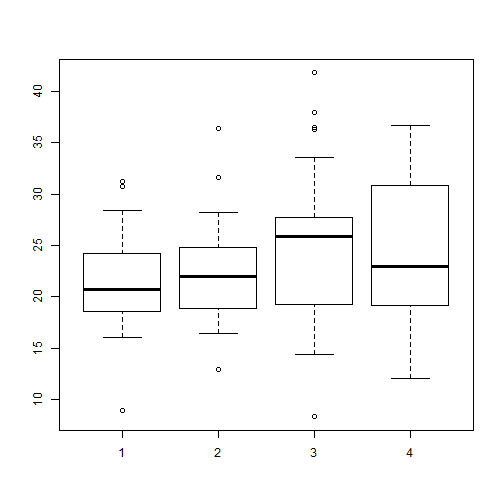Now we have more “outliers” for groups 1 – 3 but still none for group 4. Obviously the “outlier” classification is subjective. You'll also notice the size of the boxes didn't change. They will always capture the middle 50% of the data no matter the value of range. If we set range to 0, then the whiskers will extend to the minimum and maximum values for each group. In that case you get a plot of what is known as Tukey's Five-number summary: minimum, 25th percentile, median, 75th percentile and the maximum. In fact there's a function in R to calculate the Five-Number summary called fivenum. Here's how we can use it on our data:

tapply(y,x,fivenum)

## $1 ##  8.911472 18.533341 20.759577 24.181534 31.226286 ## ##$2
##  12.90309 18.90152 21.98459 24.84778 36.40995
##
## $3 ##  8.300651 19.281150 25.924704 27.721310 41.843672 ## ##$4
##  12.05522 19.14307 23.00494 30.84133 36.66171

boxplot(y ~ x, range=0)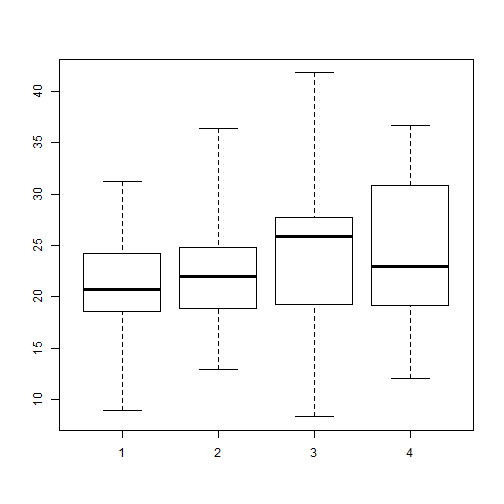A nice complement to boxplots are stripcharts. These are one-dimensional scatter plots of data. Here's out it works “out of the box”:

stripchart(y ~ x)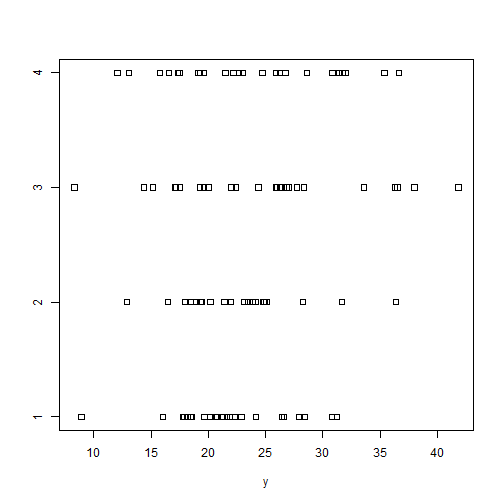I usually like to rotate stripcharts and use the familiar open circles with some “jitter”:

stripchart(y ~ x, vertical = TRUE, pch=1, method="jitter")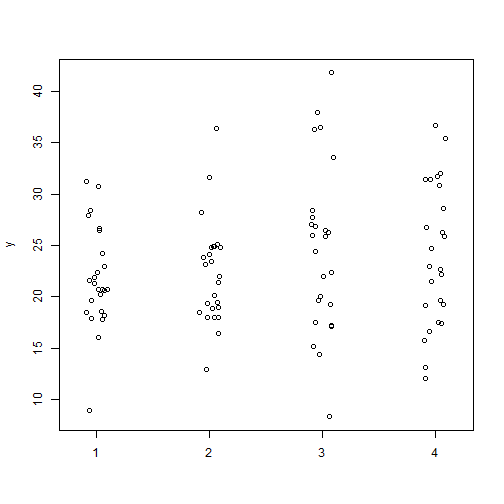Plotting stripcharts and boxplots side-by-side can be useful to visualize the spread and distribution of data. You can also calculate means and medians add them with the points function:

op <- par(mfrow=c(1,2))
means <- tapply(y,x,mean) # calculate means
medians <- tapply(y,x,median) # calculate medians
boxplot(y ~ x, ylab="y")
points(x = 1:4,y=means,pch=19) # add means
stripchart(y ~ x, vertical = TRUE, pch=1, method="jitter")
points(x = 1:4,y=means,pch=19, col="blue") # add means
points(x = 1:4,y=medians,pch=19, col="red") # add means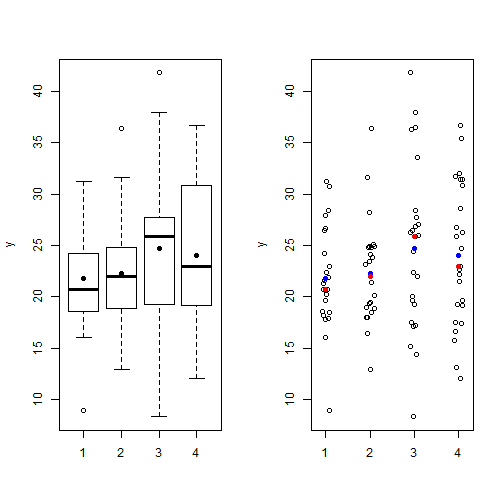par(op) # reset graphics parameters


# Poisson regression – Ch 6 of Gelman and Hill

Chapter 6 of Gelman and Hill's Data Analysis Using Regression and Multilevel/Hierarchical Models presents an interesting example of Poisson regression using data on police stops in New York. Put simply, they seek to model the count of “stop and frisks” as a function of ethnicity and precinct with number of arrests in the previous year included as an offset. While the example is fun and informative, it's not terribly easy to replicate. The R code is provided to run the regressions but the data is a little hard to locate. I was eventually able to find it and get everything to mostly work, so I thought I would blog about it in case anyone else was trying to do the same.

About the data: it turns out you can find it here in the “police” folder. In the folder is a dat file called “frisk_with_noise”. Here's one way to read it in:

url <- "http://www.stat.columbia.edu/~gelman/arm/examples/police/frisk_with_noise.dat"

##   stops  pop past.arrests precinct eth crime
## 1    75 1720          191        1   1     1
## 2    36 1720           57        1   1     2
## 3    74 1720          599        1   1     3
## 4    17 1720          133        1   1     4

dim(dat)

##  900   6


But wait. You'll notice the data set has 900 rows but the regression output in the book references n = 225. To replicate the example in the book, I'm pretty sure we need to aggregate over precinct and eth, like so:

stops <- aggregate(cbind(stops, past.arrests) ~ eth + precinct, data=dat, sum)


Using cbind() on stops and past.arrests allows us to sum both stops and past.arrests over all combinations of eth and precinct. Now we're ready to run the code provided in the book, which can also be found here. Here's one of the models they fit:

library(arm) # for the display() function
fit.2 <- glm (stops ~ factor(eth), data=stops, family=poisson, offset=log(past.arrests))
display(fit.2)

## glm(formula = stops ~ factor(eth), family = poisson, data = stops,
##     offset = log(past.arrests))
##              coef.est coef.se
## (Intercept)  -0.59     0.00
## factor(eth)2  0.07     0.01
## factor(eth)3 -0.16     0.01
## ---
##   n = 225, k = 3
##   residual deviance = 45437.4, null deviance = 46120.3 (difference = 682.9)


That works, but our output doesn't match the book's output! What's going on? Actually the explanation is in the title of the data file: “frisk_with_noise”. Also notice how I skipped the first 6 lines of the file when reading it in to R. Here's the first line of what I skipped:

stop and frisk data (with noise added to protect confidentiality)


So noise was apparently added after publication of the book to protect confidentiality. I guess we're not supposed to see which precincts are making the most stops of certain ethnicities? Oh well, at least we can run the code now.

We can see fitted values using the fitted() function. Here are the first 10 fitted values compared to their observed values:

cbind(observed=stops$stops[1:10], fitted=fitted(fit.2)[1:10])  ## observed fitted ## 1 202 544.2816 ## 2 102 175.7562 ## 3 81 180.0316 ## 4 132 418.2082 ## 5 144 331.8515 ## 6 71 203.6578 ## 7 752 1215.1920 ## 8 441 373.5563 ## 9 410 584.9845 ## 10 385 261.5884  Doesn't look too good to the eye, at least not the first 10. How are those fitted values calculated? Like this: $y = exp(\alpha + \beta x + log(t))$ where t is the offset, in this case, past arrrests. So to calculate the fitted value for precinct 1, ethnicity = 1 (black), and 980 arrests in the previous year, we do the following: exp(coef(fit.2) + log(980))  ## (Intercept) ## 544.2816  # or equivalently 980*exp(coef(fit.2))  ## (Intercept) ## 544.2816  # compare to R's fitted value fitted(fit.2) == exp(coef(fit.2) + log(980))  ## 1 ## TRUE  Plotting standardized residuals versus fitted values can reveal overdispersion. That's what Gelman and Hill do in figure 6.1. Below I reproduce it. First I fit a new model that includes precinct as a predictor. The figure on the left plots raw residuals versus fitted values. This is of limited use since we expect variance to increase with larger fitted values in a Poisson distribution. In other words we wouldn't expect to see a constant scatter about 0 as we would in OLS regression. The figure on the right, however, uses standardized residuals which have a mean of 0 and standard deviation of 1. If the Poisson model is true, we would expect to see most residuals falling within 2 standard deviations from 0. Many residuals falling beyond this range reveal overdispersion. And indeed that's what we see here. fit.3 <- glm (stops ~ factor(eth) + factor(precinct), data=stops, family=poisson, offset=log(past.arrests)) par(mfrow=c(1,2)) pv <- fitted(fit.3) r <- (stops$stops - fitted(fit.3))
plot(pv, r, pch=20, ylab="raw residuals", xlab="predicted value")
abline(h=0)
sr <- (stops\$stops - fitted(fit.3))/sqrt(fitted(fit.3))
plot(pv, sr, pch=20, ylab="standardized residuals", xlab="predicted value")
abline(h=c(-2,0,2),lty=c(2,1,2))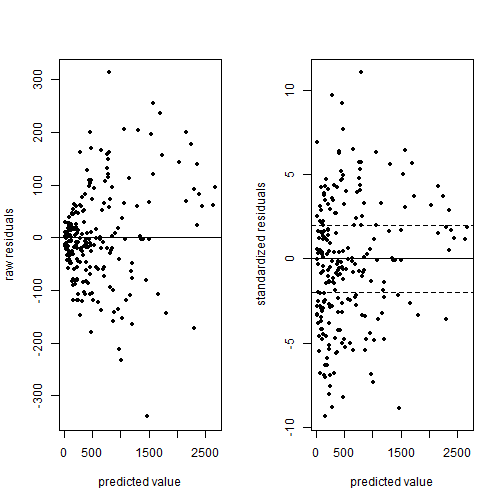par(mfrow=c(1,1))


To correct for overdisperson in R you can repeat the code for fit.3 above with family = quasipoisson. This corrects the standard errors of the regression coefficients by making them larger.